# Archimedean Solids

The Archimedean solids are symmetric semi-regular polyhedra made of two or three regular polygons that meet at identical vertices. There are 13 Archimedean solids plus two mirror image forms.

Five of these are made by taking a Platonic Solid and truncating (cutting off) a regular triangular, square, or pentagonal pyramid from each corner.

Here the vertex configuration refers to the type of regular polygons that meet at any given vertex. For example, a vertex configuration of (4.6.8) means that a square, hexagon, and octagon meet at a vertex (with the order taken to be clockwise around the vertex).

Images are from Wikipedia.org.

Name
(Vertex configuration)
Shape Faces Edges Vertices
truncated tetrahedron
(3.6.6)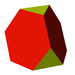8 4 triangles
4 hexagons
18 12
cuboctahedron
(3.4.3.4)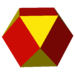14 8 triangles
6 squares
24 12
truncated cube
(3.8.8)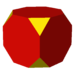14 8 triangles
6 octagons
36 24
truncated octahedron
(4.6.6)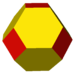14 6 squares
8 hexagons
36 24
small rhombicuboctahedron
(3.4.4.4 )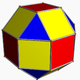26 8 triangles
18 squares
48 24
great rhombicuboctahedron
(4.6.8)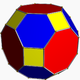26 12 squares
8 hexagons
6 octagons
72 48
snub cube
(2 chiral forms)
(3.3.3.3.4)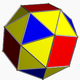38 32 triangles
6 squares
60 24
icosidodecahedron
(3.5.3.5)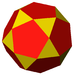32 20 triangles
12 pentagons
60 30
truncated dodecahedron
(3.10.10)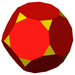32 20 triangles
12 decagons
90 60
Truncated icosahedron
(5.6.6 )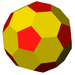32 12 pentagons
20 hexagons
90 60
small rhombicosidodecahedron
(3.4.5.4)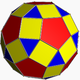62 20 triangles
30 squares
12 pentagons
120 60
great rhombicosidodecahedron
(4.6.10)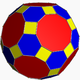62 30 squares
20 hexagons
12 decagons
180 120
snub dodecahedron
or snub icosidodecahedron
(2 chiral forms)
(3.3.3.3.5)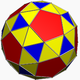92 80 triangles
12 pentagons
150 60